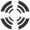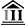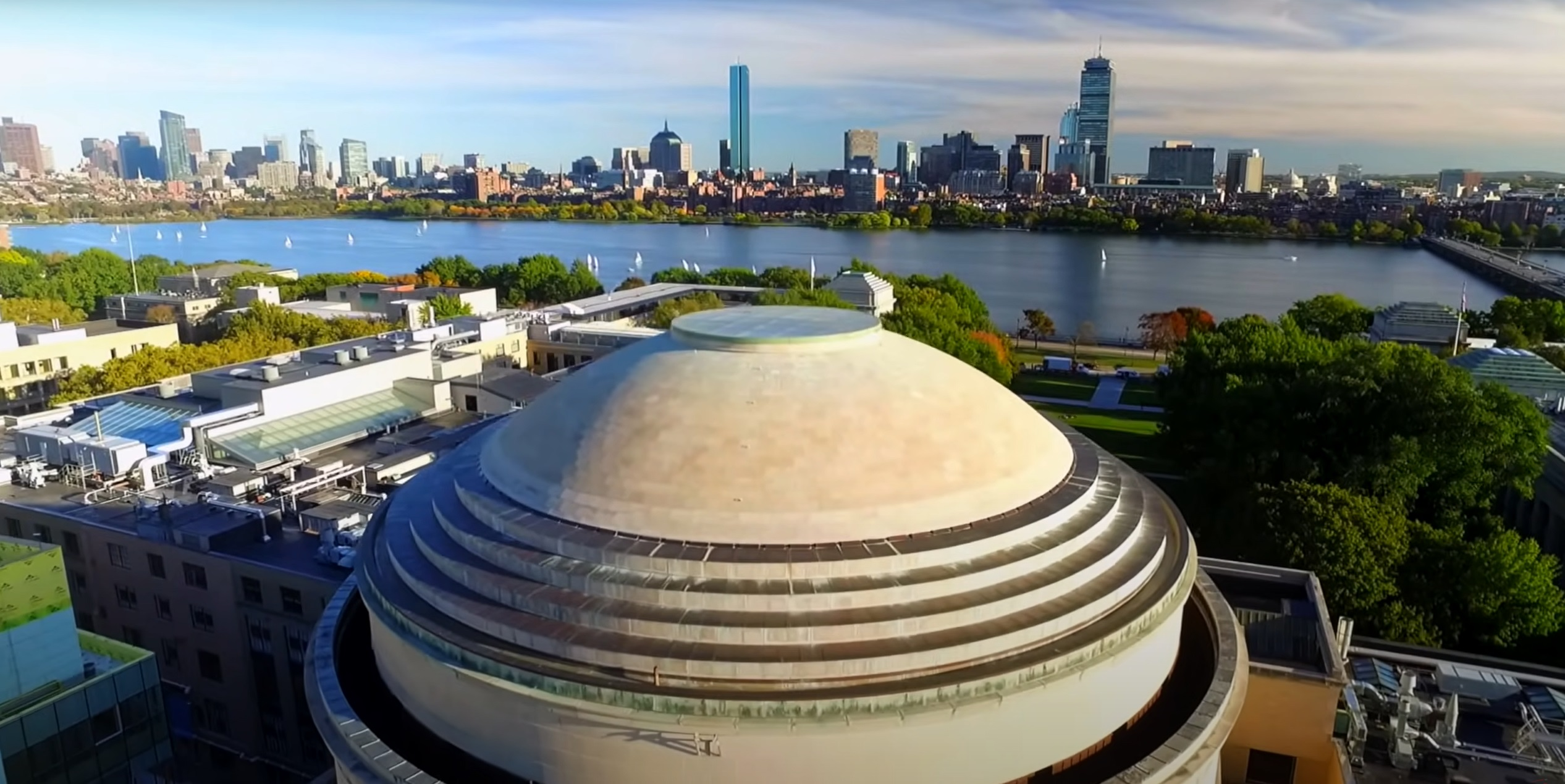# Drivers facing the yellow-light-dilemma## Drivers facing the yellow-light-dilemma

Stochastic hybrid models for predicting the behavior of drivers facing the yellow-light-dilemma

University of Michigan

Massachusetts Institute of Technology

Abstract:  We address the problem of predicting whether a driver facing the yellow-light-dilemma will cross the intersection with the red light. Based on driving simulator data, we propose a stochastic hybrid system model for driver behavior. Using this model combined with Gaussian process estimation and Monte Carlo simulations, we obtain an upper bound for the probability of crossing with the red light. This upper bound has a prescribed confidence level and can be calculated quickly on-line in a recursive fashion as more data become available. Calculating also a lower bound we can show that the upper bound is on average less than 3% higher than the true probability. Moreover, tests on driving simulator data show that 99% of the actual red light violations, are predicted to cross on red with probability greater than 0.95 while less than 5% of the compliant trajectories are predicted to have an equally high probability of crossing. Determining the probability of crossing with the red light will be important for the development of warning systems that prevent red light violations.